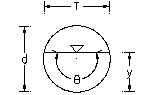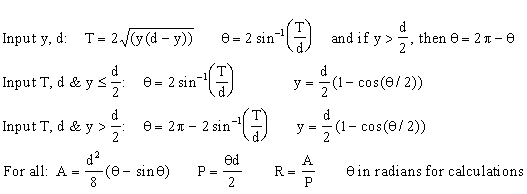Open Channel Flow

Open channel flow. Compute hydraulic radius, wetted perimeter, and area for circular culvert

 Select calculation: Enter d, y Enter d, T. Pipe ≤ half full Enter d, T. Pipe > half full Diameter, d (L): © 2014 LMNO Engineering, Depth, y (L): Research, and Software, Ltd. Top Width, T (L): http://www.LMNOeng.com Area, A (L2): Wetted Perimeter, P (L): Hydraulic Radius, R (L): Angle, θ (degrees):

Open channel flow units: (L) means that the variable has units of length (e.g. meters). (L2) means that the variable has units of length squared (e.g. m2). For instance, enter d, y, and T all in meters or all in inches or all in feet.

Open Channel Flow Culvert Geometry Diagram and Equations:Top width, T, is the top width of the water in the pipe. T=0 if the pipe is empty or full. T=d if the pipe is half full.Open channel flow circular culvert geometry calculation does not check for unreasonable inputs. For open channel flow, please enter 0<y<d, 0<T<d, and d>0.

LMNO Engineering, Research, and Software, Ltd.
7860 Angel Ridge Rd.   Athens, Ohio 45701  USA   Phone: (740) 592-1890
LMNO@LMNOeng.com    http://www.LMNOeng.com

To:

Manning Equation Calculator

Culvert Design using Inlet and Outlet Control

Circular Culverts using Manning Equation

Unit Conversions

Other information:

Register

Discussion and References